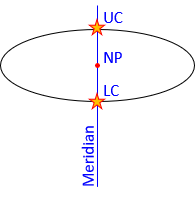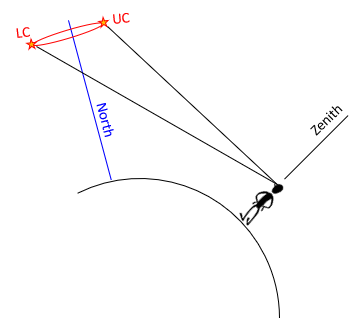#### Problem (1)

A 6 inch focal length aerial camera was used to take vertical photography of relatively flat terrain having an average elevation of 870 feet. What is the scale of the photography if the plane was flying at an altitude of 2200 feet MSL? Express as a representativre fraction, 1 inch = XXXX feet, to nearest 10 ft.
Answer1 inch = 220 feet

#### Problem (2)

T     F     At culmination, Polaris is due north of the observer.

True; At upper and lower culmination, Polaris is on the observer's meridian.#### Problem (3)

T     F     An astronomic azimuth is the same as a geodetic azimuth.

False; Astronomic is referenced to the gravity (geoid) while geodetic is referenced to the ellpsoid. The difference between the two is the Laplace Correction.

#### Problem (4)

An orthometric height is measured with respect to the:

(a) geoid
(b) ellipsoid
(c) spheroid

(a) geoid

#### Problem (5)

A grid factor of 0.99995 is the same as a distortion of 1/____________ .1/20,000

#### Problem (6)

The maximum design distortion (ellipsoid to grid) for the original State Plane Coordinate systems is 1/____________ .

1/10,000

#### Problem (7)

T    F     An azimuth determination using Polaris is generally more accurate than one using the Sun.
Answer True; Polaris is a much smaller sight target and its apparent motion is considerably less than the Sun's. Both of these reduce pointing and timing errors.

#### Problem (8)

What are the lengths (to 0.01') of the sides of the following triangular parcel?Area of a triangleCompute interior angles from the bearingsLaw of Sines to solve for side eSubstitute eqn (2) into eqn (1) and sort terms to solve side bInsert values and solve for side bLaw of Sines with numbersUse Law of Sines to solve sides e and d594.823ft, 389.209ft, 560.778ft

#### Problem (9)

T     F     On a pair of overlapping photographs, parallax is parallel with the flight direction.
Answer  True; Parallax is the photo-to-photo image shift based on changed photo position. Since the photo positions change based on flight direction, images shift parallel with flight direction.#### Problem (10)

T     F     At lower culmination Polaris is between the north pole and the observer's zenith.
Answer False; At lower culmination (LC), the star is below the Pole which places it on the other side of the Pole from the user.#### Problem (11)

The dimensions of a rectangular field are142.1 ft by 93.6 ft. What is the area of the field expressed to a reasonable level of accuracy:

Part (a) in square yards?
Part (b) in acres?The original dimensions had 4 and 3 significant figures. A product can have only as many sig fig as the least sig fig of the numbers multiplied. The conversion factors used here are exact so do not affect answer accuracy. The areas can only have 3 sig fig.
1480 yd2; 0.305ac

#### Problem (12)

The magnetic bearing of a property line was recorded as S 88º42' E in 1875. The magnetic bearing of the line today is S 84º10' E with a current declination of 1º10' W.

Part (a) What was the declination in 1875?
Part (b) What is the true bearing of the line?Part (a)Part (b)#### Problem (13)

The bearing of line L to Q is S 23°15' W. The deflection angle at Q to M is 48°15' L. What is the bearing of the line Q to M?#### Problem (14)

An incoming +5.0% grade intersects an outgoing -4.0% grade at station 37+45.00 with an elevation of 1410.00 feet. A 800.00 foot long vertical curve will be fit between the two grade lines. What is the curve elevation at station 38+00.00?Elev at Stat 38+00 is 1401.105 ft

#### Problem (15)

The PI of an alignment is at station 45+24.00 with a deflection angle of 55º30'00” left. A 1000.00 ft radius curve is to be fit between the two horizontal tangents placing the PC at station at 39+97.87. At the PC, what are the deflection angle from the PC and the radial chord to curve station 44+00.00?To 44+00: 11°31'13" deflection left at 339.43 ft

#### Problem (16)

A survey crew using a TSI didn't know the temperature and pressure in the field so they set a 0 ppm correction in the instrument. They measured and recorded a horizontal distance as 823.42 feet. Later in the office, they determined the correction should have been -18 ppm. What is the corrected distance? Compute to 0.001'.
Answer823.405 ft

#### Problem (17)

A reverse curve must be fit to the tangents shown below:Point Station PC1 21+83.05 PI1 27+35.80 PI2 38+43.05

What are the radii of the two curves which fit this alignment? Compute to 0.01 ft.

Answer1790.41 ft and 1344.35 ft

#### Problem (18)

T     F     The voulme determined by Average End Area method between two different cross sections is too small.
Answer  False; The Average End Area Formula generally gives volumes that are too large.

#### Problem (19)

Given the traverse data, adjust the latitude and departure of line B-C by the Compass Rule (aka, Bowditch Rule).Point Int angle A 75°36'06" B 124°37'48" C 71°24'36" D 88°21'30" sum 180°00'00"
 Unadjusted Line Azimuth Length Lat Dep A-B 37°42'06" 330.09 +261.169 +201.866 B-C 93°04'18" 401.56 -21.518 +400.893 C-D 201°39'42" 458.85 -426.446 -169.373 D-A 293°18'12" 471.36 +186.470 -432.908 sums: -0.325 +0.568Need total distance. Add traverse sides lengths: 1661.86 ft#### Problem (20)

The scale of a map is  1 in = 200 ft. What is the map scale expressed as a dimensionless ratio?
Answer  To convert an equaivalence to a ratio, both sides must have the same units.
Convert 200 ft to inchesScale is 1 inch = 2400 inches or 1:2400.